Courses

# Chemistry- CY 2012 GATE Paper (Practice Test)

## 65 Questions MCQ Test GATE Past Year Papers for Practice (All Branches) | Chemistry- CY 2012 GATE Paper (Practice Test)

Description
This mock test of Chemistry- CY 2012 GATE Paper (Practice Test) for GATE helps you for every GATE entrance exam. This contains 65 Multiple Choice Questions for GATE Chemistry- CY 2012 GATE Paper (Practice Test) (mcq) to study with solutions a complete question bank. The solved questions answers in this Chemistry- CY 2012 GATE Paper (Practice Test) quiz give you a good mix of easy questions and tough questions. GATE students definitely take this Chemistry- CY 2012 GATE Paper (Practice Test) exercise for a better result in the exam. You can find other Chemistry- CY 2012 GATE Paper (Practice Test) extra questions, long questions & short questions for GATE on EduRev as well by searching above.
QUESTION: 1

Solution:
QUESTION: 2

Solution:
QUESTION: 3

### Which one of the following options is the closest in meaning to the word given below? Latitude

Solution:
QUESTION: 4

Choose the most appropriate word from the options given below to complete the following
sentence:
Given the seriousness of the situation that he had to face, his ___ was impressive.

Solution:
QUESTION: 5

Choose the most appropriate alternative from the options given below to complete the following
sentence:
If the tired soldier wanted to lie down, he ___ the mattress out on the balcony.

Solution:
QUESTION: 6

Q. 6 - Q. 10 carry two marks each.

Q

One of the legacies of the Roman legions was discipline. In the legions, military law prevailed
and discipline was brutal. Discipline on the battlefield kept units obedient, intact and fighting,
even when the odds and conditions were against them.

Which one of the following statements best sums up the meaning of the above passage?

Solution:
QUESTION: 7

A and B are friends. They decide to meet between 1 PM and 2 PM on a given day. There is a
condition that whoever arrives first will not wait for the other for more than 15 minutes. The
probability that they will meet on that day is

Solution:
QUESTION: 8

The data given in the following table summarizes the monthly budget of an average household.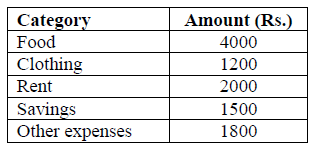The approximate percentage of the monthly budget NOT spent on savings is

Solution:
QUESTION: 9

There are eight bags of rice looking alike, seven of which have equal weight and one is slightly
heavier. The weighing balance is of unlimited capacity. Using this balance, the minimum number
of weighings required to identify the heavier bag is

Solution:
QUESTION: 10

Raju has 14 currency notes in his pocket consisting of only Rs. 20 notes and Rs. 10 notes. The total
money value of the notes is Rs. 230. The number of Rs. 10 notes that Raju has is

Solution:
QUESTION: 11

Q. 11 – Q. 35 carry one mark each

Q.

In the proton decoupled 13C NMR spectrum of 7-norbornanone, the number of signals obtained is

Solution:
QUESTION: 12

I dentify the most probable product in the given reaction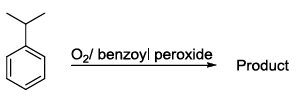Solution:
QUESTION: 13

I n the cyclization reaction given below, the most probable product formed is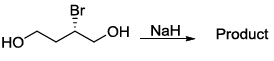Solution:
QUESTION: 14

If Δy and Δpy are the uncertainties in the y-coordinate and the y component of the momentum of a
particle respectively, then, according to uncertainty principle ΔyΔpy is ( h=2/2π) and h is Planck’s
constant)

Solution:
QUESTION: 15

The average length of a typical α-helix comprised of 10 amino acids is

Solution:
QUESTION: 16

Number of thymine residues in a 5000 kb DNA containing 23% guanine residues is

Solution:
QUESTION: 17

S hown below is a Hammett plot obtained for the reaction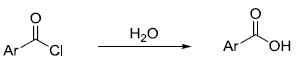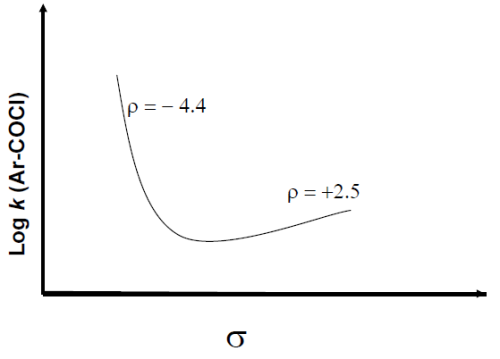The change in slope of the plot indicates that

Solution:
QUESTION: 18

The ratio of relative intensities of the two molecular ion peaks of methyl bromide (CH3Br) in the
mass spectrum is

Solution:
QUESTION: 19

A disaccharide that will not give Benedict’s test and will not form osazone is

Solution:
QUESTION: 20

Choose the allowed transition

Solution:
QUESTION: 21

T he angular part of the wavefunction for the electron in a hydrogen atom is proportional to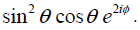. The values of the azimuthal quantum number ( l ) and the magnetic quantum
number (m ) are, respectively

Solution:
QUESTION: 22

L et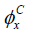and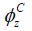denote the wavefunctions of the 2px and 2pz orbitals of carbon, respectively, and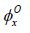and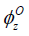represent the wavefunctions of the 2px and 2pz orbitals of oxygen, respectively. If  c1 and c2 are constants used in linear combinations and the CO molecule is oriented along the z axis, then, according to molecular orbital theory, the π-bonding molecular orbital has a wave function given by

Solution:
QUESTION: 23

The bond that gives the most intense band in the infrared spectrum for its stretching vibration is

Solution:
QUESTION: 24

I f xA and xB are the respective mole fractions of A and B in an ideal solution of the two and TA, TB,
T are the fusion temperatures of pure A, pure B and the ideal solution respectively, then

Solution:
QUESTION: 25

For a reaction involving two steps given below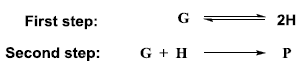assume that the first step attains equilibrium rapidly. The rate of formation of P is proportional to

Solution:
QUESTION: 26

A metal chelate that can be used for separation and quantitative analysis of aluminium ions by gas
chromatography is

Solution:
QUESTION: 27

The enthalpies of hydration of Ca2+, Mn2+ and Zn2+ follow the order

Solution:
QUESTION: 28

The number of terminal carbonyl groups present in Fe2(CO)9 is

Solution:
QUESTION: 29

Among the following substituted silanes, the one that gives cross-linked silicone polymer upon
hydrolysis is

Solution:
QUESTION: 30

The plot of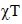versus T (where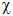is molar magnetic susceptibility and T is the temperature) for a
paramagnetic complex which strictly follows Curie equation is

Solution:
QUESTION: 31

A mong the following donors, the one that forms most stable adduct with the Lewis acid B(CH3)3 is

Solution:
QUESTION: 32

T he complex with inverse-spinel structure is

Solution:
QUESTION: 33

T he IUPAC nomenclature of Na[PCl6] is

Solution:
QUESTION: 34

An intermediate formed during the hydroformylation of olefins using Co2(CO)8 as catalyst is

Solution:
QUESTION: 35

T he order of polarity of NH3, NF3 and BF3 is

Solution:
QUESTION: 36

Q. 36 to Q. 65 carry two marks each.

Q.

F rom a carboxymethyl-cellulose column at pH 6.0, arginine, valine and glutamic acid will elute in
the order

Solution:
QUESTION: 37

S ymmetry operations of the four C2 axes perpendicular to the principal axis belong to the same
class in the point group(s)

Solution:
QUESTION: 38

A t 298 K, the EMF of the cell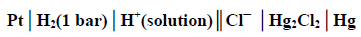is 0.7530 V. The standard potential of the calomel electrode is 0.2802 V. If the liquid junction
potential is zero, the pH of the solution is

Solution:
QUESTION: 39

T he wavefunction of a 1-D harmonic oscillator between x =+∞  and x =- ∞  is given by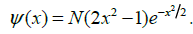The value of N that normalizes the function ψ (x) is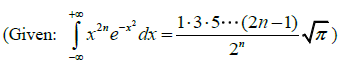Solution:
QUESTION: 40

Consider the reaction

H2 + C2H4 → C2H

The molecular diameters of H2 and C2H4 are 1.8 Å and 3.6 Å respectively. The pre-exponential
factor in the rate constant calculated using collision theory in m3 (mole)-1 s-1  is approximately
(For this reaction at 300 K,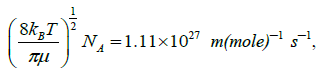where the symbols have their usual meanings)?

Solution:
QUESTION: 41

The molecular partition function of a system is given by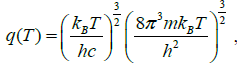where the symbols have their usual meanings. The heat capacity at constant volume for this system is

Solution:
QUESTION: 42

C onsider the phase diagram given below.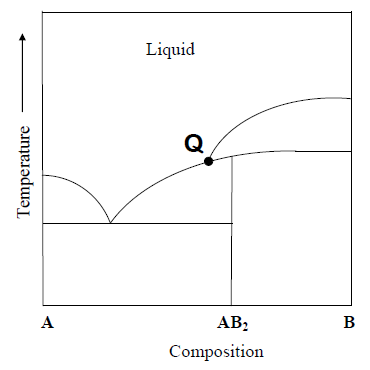At the intersection point Q the phases that are in equilibrium are

Solution:
QUESTION: 43

I dentify the product from the following reaction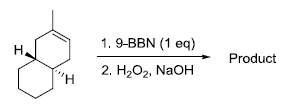(9-BBN = 9-Borabicyclo[3•3•1]nonane)

Solution:
QUESTION: 44

The product from the following reaction is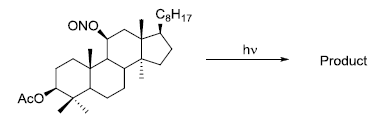Solution:
QUESTION: 45

T he acid catalyzed cyclization of 5-ketodecan-1,9-diol is given below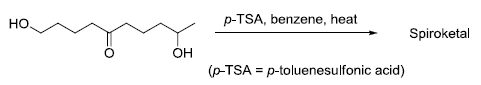The most predominant spiroketal is

Solution:
QUESTION: 46

For a face centered cubic lattice, the Miller indices for the first Bragg’s peak (smallest Bragg angle)
are

Solution:
QUESTION: 47

For the titration of a 10 mL (aq) solution of CaCO3, 2 mL of 0.001 M Na2EDTA is required to
reach the end point. The concentration of CaCO3 (assume molecular weight of CaCO3 = 100) is

Solution:
QUESTION: 48

In the reaction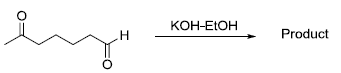the product formed is

Solution:
QUESTION: 49

In the reaction given below, identify the product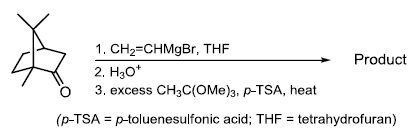(Correct Answer will be updated soon , Temoprary marked A)

Solution:
QUESTION: 50

C onsider the following pairs of complexes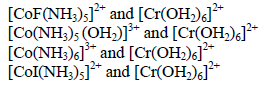The electron transfer rate will be fastest in the pair

Solution:
QUESTION: 51

The extent of Mössbauer quadrupole splitting of iron follows the order

Solution:
QUESTION: 52

Hemoglobin is an oxygen carrying protein. The correct statement about oxy-hemoglobin is that

Solution:
QUESTION: 53

If a mixture of NaCl, conc. H2SO4 and K2Cr2O7 is heated in a dry test tube, a red vapour (P) is
formed. This vapour (P) dissolves in aqueous NaOH to form a yellow solution, which upon
treatment with AgNO3 forms a red solid (Q). P and Q are, respectively

Solution:
QUESTION: 54

For the following reaction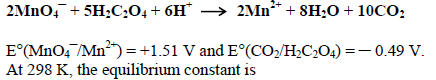Solution:
QUESTION: 55

The ground states of high-spin octahedral and tetrahedral Co(II) complexes are, respectively

Solution:
QUESTION: 56

The INCORRECT statement about Zeise’s salt is

Solution:
QUESTION: 57

T he number of possible isomers for the square planar mononuclear complex [(NH3)2M(CN)2] of a
metal M is

(Correct Answer will be updated soon, Temprary marked A)

Solution:
QUESTION: 58

Common Data for Questions 58 and 59:

Consider the reaction sequence shown below: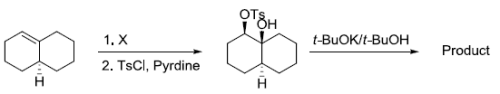TsCl = p-toluenesulfonyl chloride

Q.

T he oxidant X used in step 1 is

Solution:
QUESTION: 59

Consider the reaction sequence shown below:Q.

The product is

Solution:
QUESTION: 60

Common Data for Questions 50 and 51:

Consider the E1 reaction of tert-amyl halides from the energy profile given below.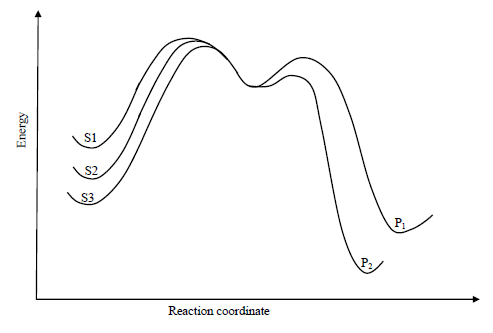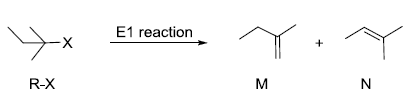Q.

In the above reaction, X = Cl, Br or I. Based on the graph, identify the alkyl halides (R-X) as S1,
S2 and S3

Solution:
QUESTION: 61

Common Data for Questions 50 and 51:

Consider the E1 reaction of tert-amyl halides from the energy profile given below.Q.

I dentify product P1 and its yield relative to P2

Solution:
QUESTION: 62

A 20491 cm-1 laser line was used to excite oxygen molecules (made of 16O only) to obtain the rotational Raman spectrum. The resulting rotational Raman spectrum of oxygen molecule has the first Stokes line at 20479 cm-1

Q.

The rotational constant (usually denoted as B) for the oxygen molecule is

Solution:
QUESTION: 63

A 20491 cm-1 laser line was used to excite oxygen molecules (made of 16O only) to obtain the rotational Raman spectrum. The resulting rotational Raman spectrum of oxygen molecule has the first Stokes line at 20479 cm-1

Q.

The next rotational Stokes line is expected at

Solution:
QUESTION: 64

Statement for Linked Answer Questions 64 and 65: Hückel molecular orbital theory can be applied to
CH2-CH-CH2

Q.

The secular determinant (where α , β and E have their usual meanings) is given by

Solution:
QUESTION: 65

Hückel molecular orbital theory can be applied to the allene radical
CH2-CH-CH2

Q.

The possible values of E are

Solution: